## Consider the use of a counter to divide an incoming clock. If our incoming clock signal is 32.768 kHz and we need a 64 Hz signal, how many b

Question

Consider the use of a counter to divide an incoming clock. If our incoming clock signal is 32.768 kHz and we need a 64 Hz signal, how many bits would our counter need to have (i.e. what is n) to divide the incoming clock correctly?

in progress 0
2 months 2021-07-28T10:49:26+00:00 1 Answers 4 views 0

The number of required bits in our  counter is 9

Explanation:

Given:

Incoming frequency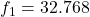kHz

Output frequency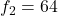Hz

From the formula of finding the number of required bits of a counter,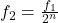Where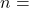number of bits,

Put all required values in above equation,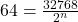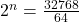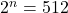Take natural log on both side,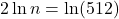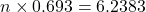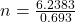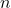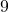Therefore, the number of required bits in our  counter is 9